IF YOU CAN SEE THIS go to /sfa/Nuprl/Shared/Xindentation_hack_doc.html

### Functionality Sequents and Well-formed Formulas - from Functions and Sequents

In Nuprl, the basic form for making assertions about the functional behavior of expressions with free variables is the Functionality Sequent, a special case of Sequents. In the degenerate form such assertions merely assert the well-formedness of expressions, indicating a type for the expression, such as "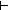(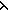x.x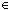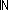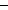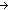" or "1+2{x: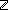| 0<x }". (Note that an expression can have many types.)
More commonly, a sequent asserts the well-formedness of an expression in free variables or under assumptions. For example, the following sequent expresses the fact that "x+x" denotes a-valued function in x:

1. x :x+xOr, considering a multi-argument function:

1. x :2. y :x+x-(y+2)Proofs of sequents often proceed by progressive decomposition of parts. See EXAMPLE

Equivalent assertions using closed forms for these function expressions would be these:(x.x+x(x,yx+x-(y+2))For any type A, and any type-valued function B(x) over xA, the following assertions are equivalent:(x.b(x))x:AB(x)

1. x : Ab(xB(x)

However, the sequent form generally is more expressive, because the sequent form

1. x : Ab(xB(x)

means that if the expression A denotes a type, then B(x) denotes a type-valued function over xA, and b(x) denotes a B(x)-valued function over A.
The purpose for this conditional is to allow us in proofs to separate the problem of showing that A denotes a type from the problem of showing other proof obligations. Suppose we want to show that "(x.b(x))x:AB(x)". This can be broken into two independent problems of showing other sequents, namely,AType, which suffices to show that A denotes a type (see Type Functionality Sequents)

and

1. x : Ab(xB(x)
.

The second of these assertions assumes for the sake of argument that A is a type, while the first of these assertions says that A is a type. Combining these justifies our original goal, namely, "(x.b(x))x:AB(x)".

(Feb 2001 - sfa )